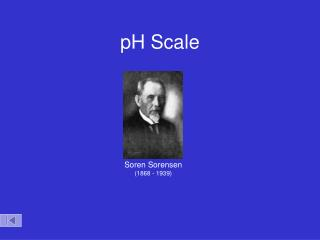# pH Scale - PowerPoint PPT PresentationDownload PresentationpH Scale

pH ScaleDownload Presentation## pH Scale

- - - - - - - - - - - - - - - - - - - - - - - - - - - E N D - - - - - - - - - - - - - - - - - - - - - - - - - - -
##### Presentation Transcript

1. pH Scale Soren Sorensen (1868 - 1939)

2. [H+] pH 10-14 14 10-13 13 10-12 12 10-11 11 10-10 10 10-9 9 10-8 8 10-7 7 10-6 6 10-5 5 10-4 4 10-3 3 10-2 2 10-1 1 100 0 1 M NaOH Ammonia (household cleaner) 7 Acid Base 0 14 Blood Pure wate Milk Acidic Neutral Basic Vinegar Lemon juice Stomach acid 1 M HCl pH Scale Zumdahl, Zumdahl, DeCoste, World of Chemistry2002, page 515

3. pH of Common Substances Timberlake, Chemistry 7th Edition, page 335

4. pH of Common Substance pH [H1+] [OH1-] pOH 14 1 x 10-14 1 x 10-0 0 13 1 x 10-13 1 x 10-1 1 12 1 x 10-12 1 x 10-2 2 11 1 x 10-11 1 x 10-3 3 10 1 x 10-10 1 x 10-4 4 9 1 x 10-9 1 x 10-5 5 8 1 x 10-8 1 x 10-6 6 6 1 x 10-6 1 x 10-8 8 5 1 x 10-5 1 x 10-9 9 4 1 x 10-4 1 x 10-10 10 3 1 x 10-3 1 x 10-11 11 2 1 x 10-2 1 x 10-12 12 1 1 x 10-1 1 x 10-13 13 0 1 x 100 1 x 10-14 14 NaOH, 0.1 M Household bleach Household ammonia Lime water Milk of magnesia Borax Baking soda Egg white, seawater Human blood, tears Milk Saliva Rain Black coffee Banana Tomatoes Wine Cola, vinegar Lemon juice Gastric juice More basic 7 1 x 10-7 1 x 10-7 7 More acidic

5. Acid – Base Concentrations 10-1 pH = 3 pH = 11 OH- H3O+ pH = 7 10-7 concentration (moles/L) H3O+ OH- OH- H3O+ 10-14 [H3O+]<[OH-] [H3O+]>[OH-] [H3O+]=[OH-] acidic solution neutral solution basic solution Timberlake, Chemistry 7th Edition, page 332

6. pH pH = -log [H1+] Kelter, Carr, Scott, Chemistry A World of Choices 1999, page 285

7. pH Calculations pH [H3O+] pH = -log[H3O+] [H3O+] = 10-pH pH + pOH = 14 [H3O+] [OH-] = 1 x10-14 pOH [OH-] pOH = -log[OH-] [OH-] = 10-pOH

8. 10x log 2nd antilog pH = - log [H+] Given: determine the [hydronium ion] pH = 4.6 choose proper equation pH = - log [H+] substitute pH value in equation 4.6 = - log [H+] multiply both sides by -1 - 4.6 = log [H+] take antilog of both sides - 4.6 = log [H+] [H+] = 2.51x10-5M Recall, [H+] = [H3O+] You can check your answer by working backwards. pH = - log [H+] pH = - log [2.51x10-5M] pH = 4.6

9. pH and pOH Calculations pH and pOH Calculations pH and pOH Calculations http://www.unit5.org/christjs/tempT27dFields-Jeff/AcidBase1.htm

10. Acid Dissociation pH = ? pH = - log [H+] H1+(aq) + A1-(aq) HA(aq) monoprotic 0.3 M 0.3 M 0.3 M pH = - log [3M] e.g. HCl, HNO3 pH = - 0.48 pH = - log [H+] 2 H1+(aq) + A2-(aq) H2A(aq) diprotic 0.3 M 0.6 M 0.3 M pH = - log [6M] e.g. H2SO4 pH = - 0.78 Given: pH = 2.1 3 H1+(aq) + PO43-(aq) polyprotic H3PO4(aq) e.g. H3PO4 ? M x M find [H3PO4] assume 100% dissociation

11. - 2.1 = log [H+] 2nd log 2nd log 7.94 x10-3M 3 Given: pH = 2.1 3 H1+(aq) + PO43-(aq) H3PO4(aq) XM 0.00794 M find [H3PO4] assume 100% dissociation Step 1) Write the dissociation of phosphoric acid Step 2) Calculate the [H+] concentration pH = - log [H+] [H+] = 10-pH 2.1 = - log [H+] [H+] = 10-2.1 - 2.1 = log [H+] [H+] = 0.00794 M 7.94 x10-3M [H+] = 7.94 x10-3M Step 3) Calculate [H3PO4] concentration Note: coefficients (1:3) for (H3PO4 : H+) = 0.00265 M H3PO4

12. How many grams of magnesium hydroxide are needed to add to 500 mL of H2O to yield a pH of 10.0? Mg2+ OH1- Step 1) Write out the dissociation of magnesium hydroxide Mg(OH)2 Mg(OH)2(aq) Mg2+(aq) + 2 OH1-(aq) 0.5 x10-4 M 5 x10-5 M 1 x10-4 M pH + pOH = 14 Step 2) Calculate the pOH 10.0 + pOH = 14 pOH = 4.0 pOH = - log [OH1-] Step 3) Calculate the [OH1-] [OH1-] = 10-OH [OH1-] = 1 x10-4 M Step 4) Solve for moles of Mg(OH)2 x = 2.5 x 10-5 mol Mg(OH)2 Step 5) Solve for grams of Mg(OH)2

13. Practice Problems - Key Practice Problems - Answer Key Practice Problems - Answer Key http://www.unit5.org/christjs/tempT27dFields-Jeff/AcidBase1.htm